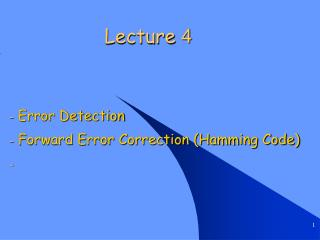DownloadDownload PresentationLecture 4

Lecture 4

Download PresentationLecture 4

- - - - - - - - - - - - - - - - - - - - - - - - - - - E N D - - - - - - - - - - - - - - - - - - - - - - - - - - -
Presentation Transcript

1. Lecture 4 Error Detection Forward Error Correction (Hamming Code)

2. 1- Error Detection 1.1 Parity check 1.2 Two dimensional parity check 1.3 Checksum 1.4 Cyclic redundancy check

3. 1.1 Parity check - Appends a parity bit to the end of the data. 10110010 0 Even 10110010 1 Odd - It can detect all single bit errors. It can also detect burst errors, if the number of bits in error is odd.

4. 1.2 Two Dimensional Parity Check Parity check bits are calculated for each row, which is equivalent to a simple parity check bit. Parity check bits are also calculated for all columns. Both are sent along with the data.

5. 1.2 Two Dimensional Parity Check

6. 1.3 Checksum - At the sender’s end, the data is divided into segments. The segments are added using ones complement arithmetic to get the sum. The sum is complemented to get the checksum which is sent along with the data segments. At the receiver, the received segments are added using ones complement arithmetic to get the sum. If the complement of the sum is zero, the received data is accepted.

7. 1.3 Checksum Ex: Find the checksum at the sender and receiver for the following sequence: 10110011 10101011 01011010 11010101 10110011 10101011 __________ 01011110 1 _______________ 01011111 01011010 _______________ 10111001 11010101 ______________ 10001110 1 _____________ 10001111 01110000 ________________ Sum: 11111111 Checksum: 00000000 10110011 10101011 __________ 01011110 1 _______________ 01011111 01011010 _______________ 10111001 11010101 ______________ 10001110 1 _____________ sum: 10001111 Checksum: 01110000

8. 1.4 Cyclic Redundancy Check • The sender generates a bit sequence known as a frame check sequence (FCS), so that the resulting frame consisting of the original data followed by this FCS.- FCS is the remainder which obtained from dividing the original data M(X) by generator polynomial G(x).- The receiver divides the incoming frame by G(X), if there is no remainder the received data has no errors.

9. 1001 1001 1011 1011 1 0 1 0 0 0 0 1 0 1 1 ________ 0 0 1 0 0 0 0 0 _________ 0 1 0 0 0 0 0 0 __________ 1 0 0 0 1 0 1 1 __________ FCS 0 1 1 1 0 1 00 1 1 1 0 1 1 ________ 0 0 1 0 0 0 0 0 _________ 0 1 0 1 0 0 0 0 __________ 1 0 1 1 1 0 1 1 __________ 0 0 0 1.4 Cyclic Redundancy Check

10. Initial 1 0 1 0 0 0 0 C3 C1 C0 1010000 + + 000 001 010 101 001 010 100 011 FCS 1.4 Cyclic Redundancy Check

11. 2- Forward Error Correction • Forward Error correction (FEC) which based on the receiver only is used mainly when retransmissions cannot be requested, e.g., simplex links. • Backward error correction which based on retransmission of the frame when an error is detected is commonly used. • Hamming Code: • It can correct any single bit error • Bits in power of 2 positions (1,2,4,8,) are check bits, the rest are m data bits • Check bit forces the parity of some collection of bit “1” to be even • To see which check bits the data bit in position k contributes to, rewrite k as a sum of powers of 2. • 11=1+2+8, 29=1+4+8+16

12. EX: If a 12-bit hamming code 100110111000 arrives at receiver, is there any bit error? If so, which bit is wrong? Hamming Code 1 2 3=2+1 4 5=4+16=4+27=4+2+1 8 9=8+110=8+211=8+2+1 12=8+4 1 0 0 1 1 0 1 1 1 0 0 0 Hence bit 6 = 2+4 is wrong, so correct hamming code should be 100111111000